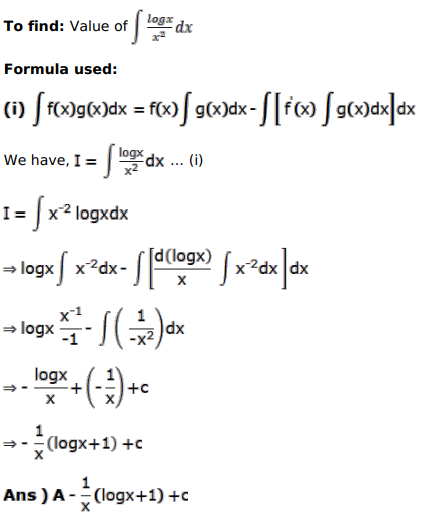# Solve this following

Question:

Mark $(\sqrt{)}$ against the correct answer in each of the following:

$\int \frac{\log x}{x^{2}} d x=?$

A. $-\frac{1}{x}(\log x+1)+C$

B. $\frac{1}{x}(\log x-1)+C$

C. $\frac{1}{\mathrm{x}}(\log \mathrm{x}+1)+\mathrm{C}$

D. none of these

Solution: